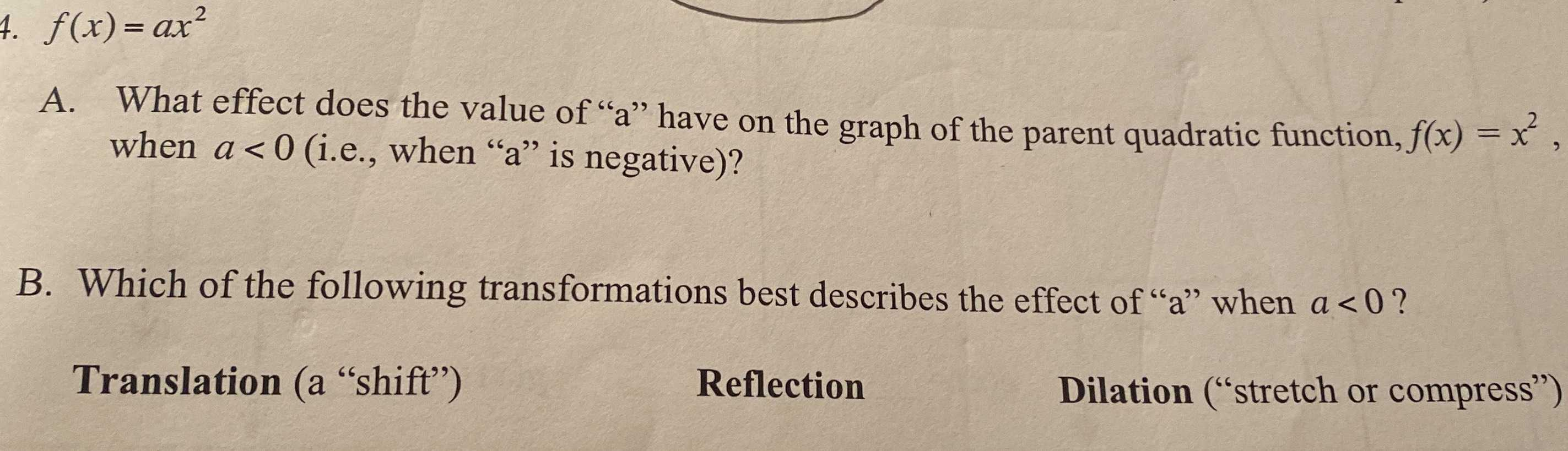### ¿Todavía tienes preguntas de matemáticas?

Pregunte a nuestros tutores expertos
Algebra
Pregunta7. $$f ( x ) = a x ^ { 2 }$$

A. What effect does the value of "a" have on the graph of the parent quadratic function, $$f ( x ) = x ^ { 2 }$$ , when $$a < 0$$ (i.e., when "a" is negative)?

B. Which of the following transformations best describes the effect of " $$a$$ " when $$a < 0$$ ?

Translation (a "shift")   Reflection  Dilation ("stretch or compress")